• ## Matlab线性拟合和非线性拟合

万次阅读 多人点赞 2019-01-23 09:25:36
线性拟合 已知如下图像的x,y坐标，x = [1.0, 1.5, 2.0, 2.5, 3.0]，y = [0.9, 1.7, 2.2, 2.6, 3.0]，如何用一条直线去拟合下列散点？ 代码： x = [1.0, 1.5, 2.0, 2.5, 3.0]'; y = [0.9, 1.7, 2.2, 2.6, 3.0]'...
线性拟合
已知如下图像的x,y坐标，x = [1.0, 1.5, 2.0, 2.5, 3.0]，y = [0.9, 1.7, 2.2, 2.6, 3.0]，如何用一条直线去拟合下列散点？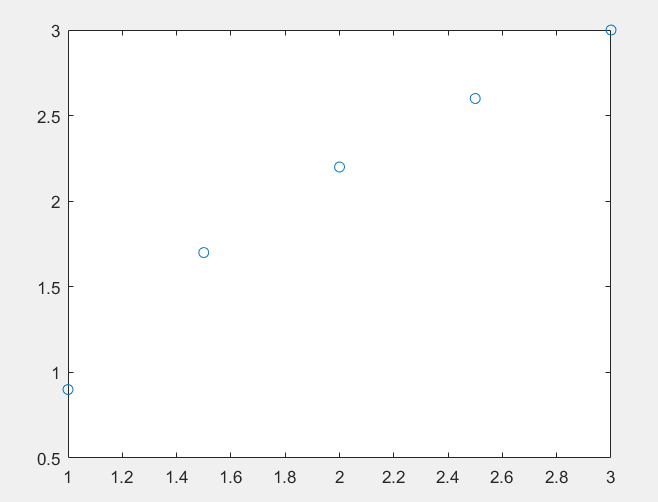代码：
x = [1.0, 1.5, 2.0, 2.5, 3.0]';
y = [0.9, 1.7, 2.2, 2.6, 3.0]';
a = polyfit(x,y,1)  % a会返回两个值，[斜率，x=0时y的值]
xi = 1:0.1:3;
yi = polyval(a,xi);
plot(x,y,'o',xi,yi);
拟合后的图像如下：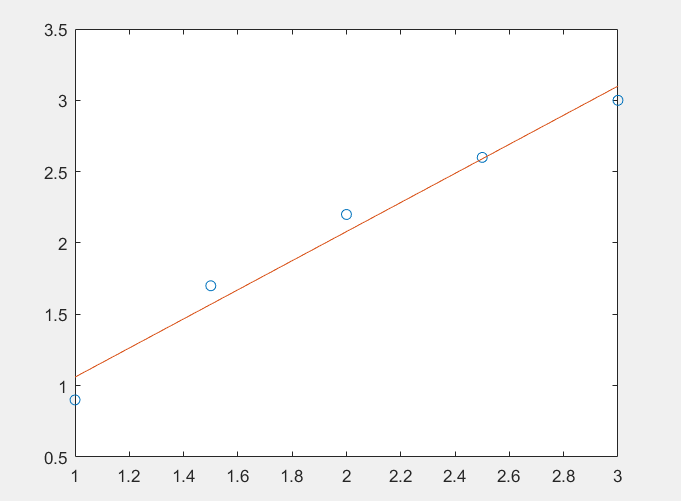非线性拟合
普通非线性拟合：已知如下图像的x,y坐标，x = [1.0, 1.5, 2.0, 2.5, 3.0]，y = [0.9, 1.7, 2.2, 2.6, 3.0]，如何用一条曲线a*x+b*sin(x)+c去拟合下列散点？代码：
x = [1.0, 1.5, 2.0, 2.5, 3.0]';
y = [0.9, 1.7, 2.2, 2.6, 3.0]';
p = fittype('a*x+b*sin(x)+c');
f = fit(x,y,p)   % f会返回a,b,c的值
plot(f,x,y);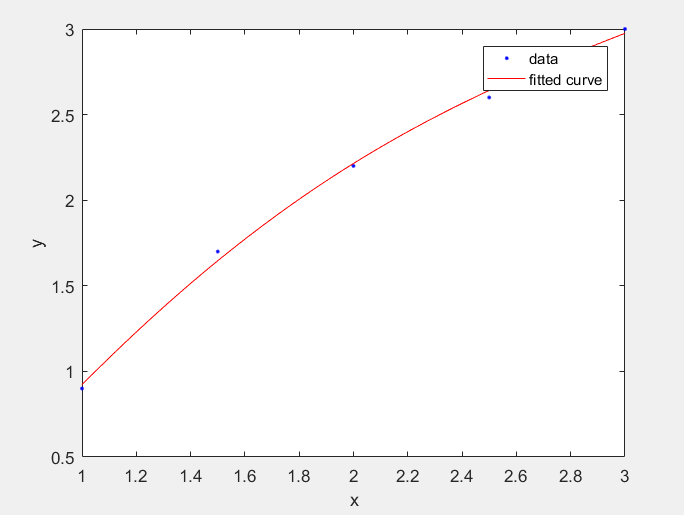指数非线性拟合：如何拟合1790-1900年美国人口指数增长模型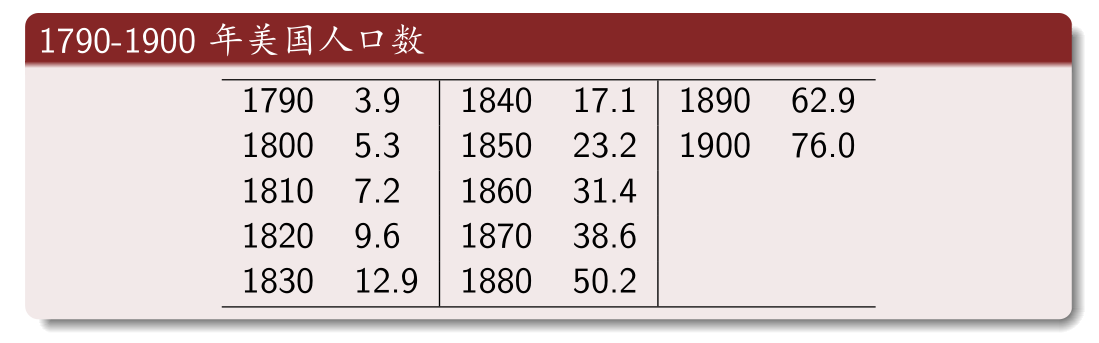思路：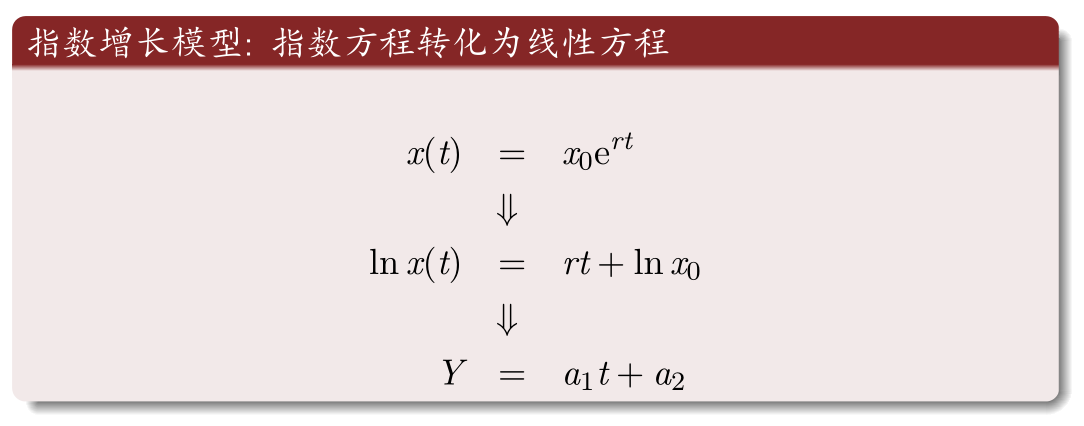实现代码：
t = 1790:10:1900;
p = [3.9 5.3 7.2 9.6 ...
12.9 17.1 23.2 31.4 ...
38.6 50.2 62.9 76.0];
Y = log(p); % Y = log(p) 返回数组p中每个元素的自然对数ln(x)
X = t;
a = polyfit(X,Y,1)
x0 = exp(a(2)); r = a(1);
ti = 1790:1900;
pti= x0*exp(r*ti);
plot(t,p,'o',ti,pti,'m')
xlabel('Year')
ylabel('Population')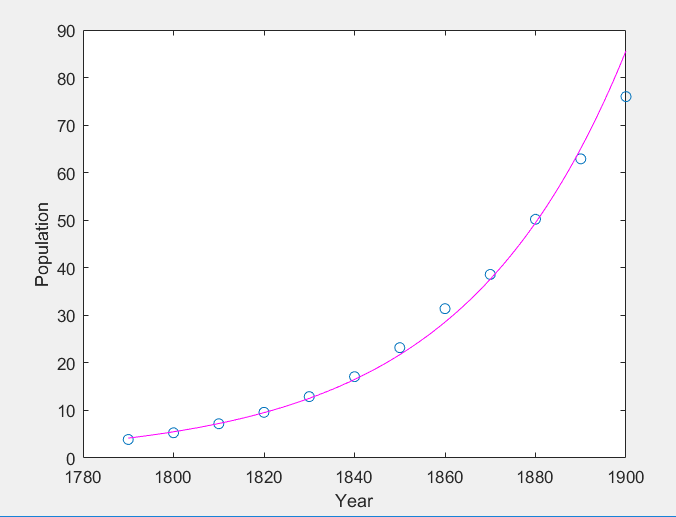对数形式的非线性拟合：
%% 对数形式非线性回归
x = [1.5, 4.5, 7.5,10.5,13.5,16.5,19.5,22.5,25.5];
y = [7.0,4.8,3.6,3.1,2.7,2.5,2.4,2.3,2.2];
plot(x, y, '*', 'linewidth', 1) % 这里的linewidth指的是散点大小
m1 = @(b,x) b(1) + b(2)*log(x);
nonlinfit1 = fitnlm(x,y,m1,[0.01;0.01])
b = nonlinfit1.Coefficients.Estimate;
Y1 = b(1,1) + b(2,1)*log(x);
hold on
plot(x, Y1, '--k', 'linewidth',2)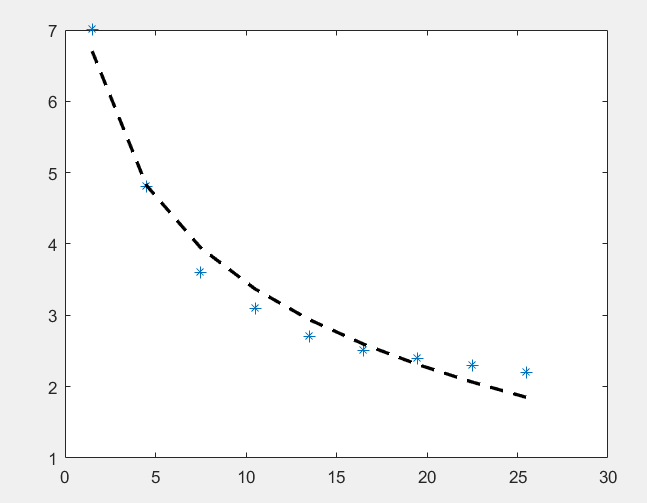展开全文• MATLAB程序分享MATLAB实现线性拟合和相关系数源程序代码-MATLAB实现线性拟合和相关系数 源程序代码.rar 程序代码见附件，拿资料请顺便顶个贴~~ 如果下载有问题，请加我 qq 1530497909，给你在线传
• MATLAB 线性拟合 ployfit函数 x = linspace(0,1,20); y = 2*x + 0.2*randn([1,20]); pf = polyfit(x,y,1); figure;plot(x,y,'o',x,polyval(t,x))
MATLAB 线性拟合

ployfit函数

x = linspace(0,1,20);
y = 2*x + 0.2*randn([1,20]);
pf = polyfit(x,y,1);
figure;plot(x,y,'o',x,polyval(t,x))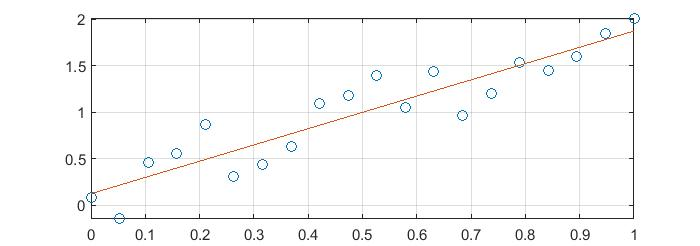展开全文• 使用Matlab进行拟合是图像处理中线条变换的一个重点内容，本文将详解Matlab中的直线拟合和曲线拟合用法。 关键函数： fittype Fit type for cu
原文地址为：
Matlab 线性拟合 & 非线性拟合

使用Matlab进行拟合是图像处理中线条变换的一个重点内容，本文将详解Matlab中的直线拟合和曲线拟合用法。
关键函数：

fittype

Fit type for curve and surface fitting
Syntax

ffun = fittype(libname)

ffun = fittype(expr)

ffun = fittype({expr1,...,exprn})

ffun = fittype(expr, Name, Value,...)

ffun= fittype({expr1,...,exprn}, Name, Value,...)

/***********************************线性拟合***********************************/
线性拟合公式：

coeff1 * term1 + coeff2 * term2 + coeff3 * term3 + ... 其中，coefficient是系数，term都是x的一次项。

线性拟合Example：
Example1: y=kx+b;
法1：

x=[1,1.5,2,2.5,3];y=[0.9,1.7,2.2,2.6,3];
p=polyfit(x,y,1);
x1=linspace(min(x),max(x));
y1=polyval(p,x1);
plot(x,y,'*',x1,y1);结果：p =    1.0200    0.0400

即y=1.0200 *x+ 0.0400法2：

x=[1;1.5;2;2.5;3];y=[0.9;1.7;2.2;2.6;3];
p=fittype('poly1')
f=fit(x,y,p)
plot(f,x,y);运行结果：

 x=[1;1.5;2;2.5;3];y=[0.9;1.7;2.2;2.6;3];
p=fittype('poly1')
f=fit(x,y,p)
plot(f,x,y);

p =

Linear model Poly1:
p(p1,p2,x) = p1*x + p2

f =

Linear model Poly1:
f(x) = p1*x + p2
Coefficients (with 95% confidence bounds):
p1 =        1.02  (0.7192, 1.321)
p2 =        0.04  (-0.5981, 0.6781)Example2:y=a*x + b*sin(x) + c
法1：

x=[1;1.5;2;2.5;3];y=[0.9;1.7;2.2;2.6;3];
EXPR = {'x','sin(x)','1'};
p=fittype(EXPR)
f=fit(x,y,p)
plot(f,x,y);

运行结果：

 x=[1;1.5;2;2.5;3];y=[0.9;1.7;2.2;2.6;3];
EXPR = {'x','sin(x)','1'};
p=fittype(EXPR)
f=fit(x,y,p)
plot(f,x,y);

p =

Linear model:
p(a,b,c,x) = a*x + b*sin(x) + c

f =

Linear model:
f(x) = a*x + b*sin(x) + c
Coefficients (with 95% confidence bounds):
a =       1.249  (0.9856, 1.512)
b =      0.6357  (0.03185, 1.24)
c =     -0.8611  (-1.773, 0.05094)

法2：

x=[1;1.5;2;2.5;3];y=[0.9;1.7;2.2;2.6;3];
p=fittype('a*x+b*sin(x)+c','independent','x')
f=fit(x,y,p)
plot(f,x,y);

运行结果：

x=[1;1.5;2;2.5;3];y=[0.9;1.7;2.2;2.6;3];
p=fittype('a*x+b*sin(x)+c','independent','x')
f=fit(x,y,p)
plot(f,x,y);

p =

General model:
p(a,b,c,x) = a*x+b*sin(x)+c
Warning: Start point not provided, choosing random start
point.
> In fit>iCreateWarningFunction/nThrowWarning at 738
In fit>iFit at 320
In fit at 109

f =

General model:
f(x) = a*x+b*sin(x)+c
Coefficients (with 95% confidence bounds):
a =       1.249  (0.9856, 1.512)
b =      0.6357  (0.03185, 1.24)
c =     -0.8611  (-1.773, 0.05094)/***********************************非线性拟合***********************************/
Example：y=a*x^2+b*x+c
法1：

x=[1;1.5;2;2.5;3];y=[0.9;1.7;2.2;2.6;3];
p=fittype('a*x.^2+b*x+c','independent','x')
f=fit(x,y,p)
plot(f,x,y);
运行结果：

p =

General model:
p(a,b,c,x) = a*x.^2+b*x+c
Warning: Start point not provided, choosing random start
point.
> In fit>iCreateWarningFunction/nThrowWarning at 738
In fit>iFit at 320
In fit at 109

f =

General model:
f(x) = a*x.^2+b*x+c
Coefficients (with 95% confidence bounds):
a =     -0.2571  (-0.5681, 0.05386)
b =       2.049  (0.791, 3.306)
c =       -0.86  (-2.016, 0.2964)法2：

x=[1;1.5;2;2.5;3];y=[0.9;1.7;2.2;2.6;3];
%use c=0;
c=0;
p1=fittype(@(a,b,x) a*x.^2+b*x+c)
f1=fit(x,y,p1)
%use c=1;
c=1;
p2=fittype(@(a,b,x) a*x.^2+b*x+c)
f2=fit(x,y,p2)
%predict c
p3=fittype(@(a,b,c,x) a*x.^2+b*x+c)
f3=fit(x,y,p3)

%show results
scatter(x,y);%scatter point
c1=plot(f1,'b:*');%blue
hold on
plot(f2,'g:+');%green
hold on
plot(f3,'m:*');%purple
hold off转载请注明本文地址：
Matlab 线性拟合 & 非线性拟合

展开全文random Mat plot 图像处理
• polyfit 结果不准 由于我的数据来源于实验，太大没法放在这里，直接放代码和图展示问题，记此笔记方便后续翻阅 [T30H_Lin, F30H_Lin] = SelectFitData(0.65,2.9,F30H, T30H); [p,S,mu] = polyfit(T30H_Lin,F30H_Lin,...
polyfit 结果不准
由于我的数据来源于实验，太大没法放在这里，直接放代码和图展示问题，记此笔记方便后续翻阅
[T30H_Lin, F30H_Lin] = SelectFitData(0.65,2.9,F30H, T30H);
[p,S,mu] = polyfit(T30H_Lin,F30H_Lin,1);  % 函数调用方式与下面有区别
T30H_Lin_Fit = T30H_Lin(1):0.1:20;
F30H_Lin_Fit = polyval(p,T30H_Lin_Fit);

plot(T30H_Lin_Fit, F30H_Lin_Fit)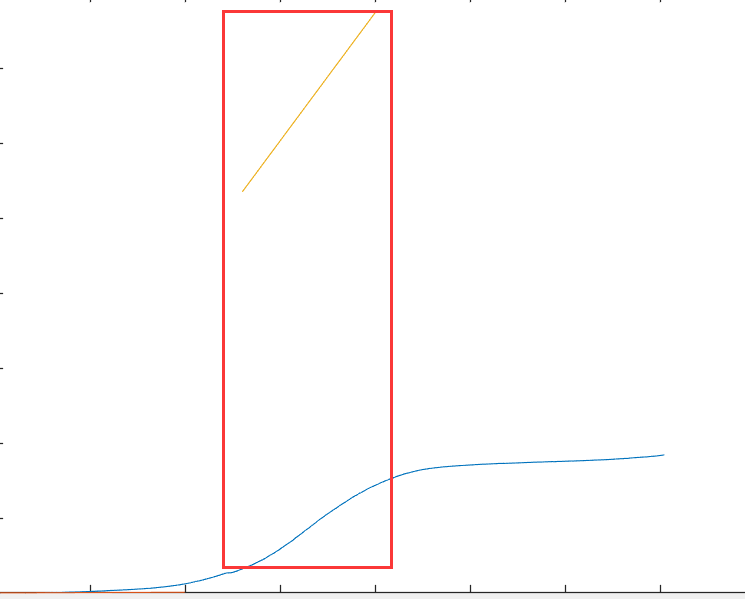[T30H_Lin, F30H_Lin] = SelectFitData(0.65,2.9,F30H, T30H);
p = polyfit(T30H_Lin,F30H_Lin,1);
T30H_Lin_Fit = T30H_Lin(1):0.1:20;
F30H_Lin_Fit = polyval(p,T30H_Lin_Fit);

plot(T30H_Lin_Fit, F30H_Lin_Fit)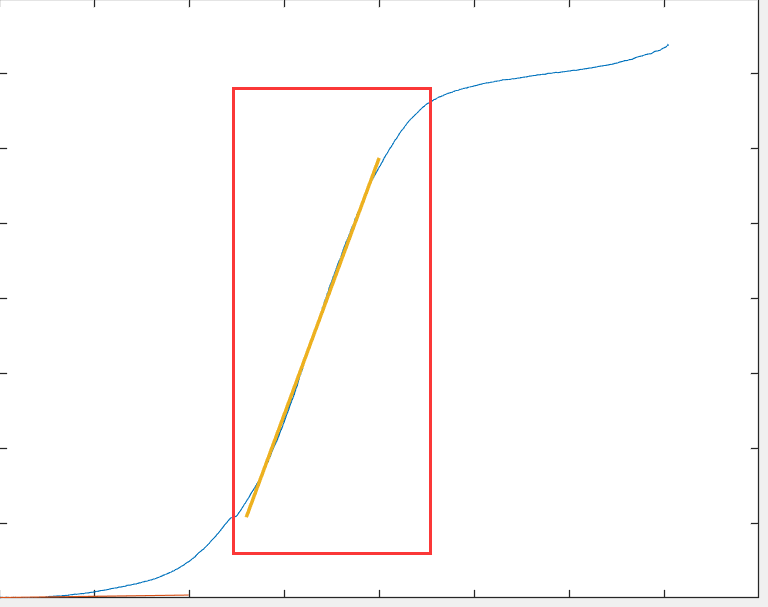结论
p = polyfit(T30H_Lin,F30H_Lin,1);
[p,S,mu] = polyfit(T30H_Lin,F30H_Lin,1);

这两种调用方式结果不一样，不应该认为返回值返回值越多越精确或者说返回值越多的肯定包含少的
展开全文polyfit
• 令我沮丧的是，我发现 matlab 的 regstats 中没有内置这个函数，或者至少我不知道如何从 regstats 中获取它。 这是计算回归统计漏斗图的一段快速代码。
• matlab线性拟合曲线代码 校电赛模拟电磁曲射炮（19年国赛H题）代码工程以及不详细且效果不太好的题解 试题 试题可在根目录找到，任务大致分为能射出、给定距离射击、给定距离和角度设计、30秒内自动搜寻标识射击、10...
• MATLAB线性拟合测定古田地区 震动持续时间震级 段刚 福建省地震局监测中心 摘要本文介绍了振动持续时间震级并利用MATLAB线性拟合来确定公式中的未知参数讨论振动持续时间震级的优缺点及适用性 主题词 振动持续时间 ...
• MATLAB 最小二乘法 线性拟合算法 线性相关系数
• MATLAB曲线拟合代码 只要改变代码中ui uo内值，便可进行所需拟合拟合后可通过workspace查看直线参数
• matlab线性代码带解释Demo---共识-最大化-树-搜索-重访 一种显着加速的全局最优共识最大化树搜索方法。 作为口头报告发表在 ICCV 2019 上。 关于 共识最大化是计算机视觉中稳健拟合的有效工具。 A* 树搜索是全局最优...
• 线性拟合之后总是需要求解决定系数R2，网上找了一下发现没啥靠谱的中文回答。还是老外的方法比较靠谱。 线性拟合求解决定系数
• 该程序能拟合得到单波段模型、两波段模型、波段比值模型和三波段模型的拟合系数。
• ## matlab线性拟合

千次阅读 2014-06-03 17:14:17
原文地址：matlab线性拟合作者：Txping7 11.1 曲线拟合 曲线拟合涉及回答两个基本问题：最佳拟合意味着什么？应该用什么样的曲线？可用许多不同的方法定义最佳拟合，并存在无穷数目的曲线。所以，从这里开始，...
• # 我们现在拟合这个y = bx+a 这里要介绍一个写好的包，regress()使用参数如下： B,BINT,R,RINT,STATS] = regress(Y,X) B：回归系数。（可以当斜率来理解） BINT：回归系数的95%置信区间，可以暂时理解为B的一个...
• LPREDICT 使用线性预测来推断数据，通常是时间序列。 注意，这与线性外推不同。 自相关系数的窗口超出数据限制以外推数据。
• 利用MATLAB中的三次样条插值法等对曲线进行拟合
• MATLAB实现最小二乘法一元线性拟合，并求出预测直线的斜率与截距。
• 首先对于函数进行分析：该函数是一个比较强的非线性函数，所以不能用一般的最小二乘法来进行拟合，如过一定要用最小二乘法，则A必须是已知的，再利用最二乘法进行拟合，附程序2.
• clear all x=[1 2 3 4 5 6 7 8 9 10]; y=[10.15 5.52 4.08 2.85 2.11 1.65 1.41 1.30 1.21 1.15]; plot(x,y,'*') hold on z=1./x;...legend('数据点','线性值') hold off
• [matlab]简单的线性拟合以及作图 说明 这是笔者第一次写博客，此文档只用于个人记录，内容来自《matlab完全自学一本通》。 相关函数 ployfit（x,y,n） —— 进行n阶线性拟合，返回多项式的系数 ployval(Pn,x) ...
• 1. 拟合多项式次数 大于或等于 X 的长度 2. 自变量 X 有重复数值或很相近的数值 3. X 可能需要变得更集中或者缩放一下 （help原始解释： Warning messages result if N is &gt;= length(...
• 不同的电压，会对应滤波器不同的频率，由于测试，不可能穷尽所有的频率，通常是在测试完一些基础频率后，用matlab线性拟合功能，完成图形的绘制。 初步测试数据如下： 初步在excel中画出来：可以看出，基本呈现...
• MATLAB 实现线性回归算法代码，运用了三种方法，包括批梯度下降，随机梯度下降，和解正规方程组，代码有注释。
• 本文来自于csdn，本文将...目的：使用一个线性函数去拟合上面这些数据； 该线性函数如下只有两个参数，利用梯度下降找出使损失值最小时，对应的两个参数值，即得到了线性函数。 算法三要素： 1）设置线性函数，即假设函...

# matlab线性拟合matlab 订阅